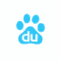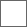• 9回复贴，共1

### 在最终战的分数出来之前，我先整理下第七轮另外三队的得分

1二宫：37
2影浦：36
3玉狛：36
4生驹：30
5东队：29
6王子：28
7铃鸣：28

1二宫：37+？（第7场）
2影浦：36+3=39
3玉狛：36
4生驹：30+？（第7场）=
5王子：28+？（第7场）+3=31+？
6东队：29+4=33
7弓场：28
8铃鸣：28

3、1+2=3、2+2=4、3+2=5

3分=第七场33分、4分=34、5分=35

2分+最终场3分=33、3分+最终场3分=34

1二宫：37+3=40
2影浦：36+3=39
3玉狛：36
4生驹：30+3=33
5王子：28+2+3=33
6东队：29+4=33
7弓场：28
8铃鸣：28

1二宫：37+4=41
2影浦：36+3=39
3玉狛：36
4生驹：30+4=34
5王子：28+3+3=34
6东队：29+4=33
7弓场：28
8铃鸣：28

1二宫：37+4=41
2影浦：36+3=39
3玉狛：36
4生驹：30+5=35
5王子：28+2+3=33
6东队：29+4=33
7弓场：28
8铃鸣：28

1二宫：37+5=42
2影浦：36+3=39
3玉狛：36
4生驹：30+4=34
5王子：28+2+3=33
6东队：29+4=33
7弓场：28
8铃鸣：28

1二宫：37+3=40
2影浦：36+3=39
3玉狛：36
4生驹：30+5=35
5王子：28+3+3=34
6东队：29+4=33
7弓场：28
8铃鸣：28

1二宫：37+4=41
2影浦：36+3=39
3玉狛：36
4生驹：30+4=34
5王子：28+3+3=34
6东队：29+4=33
7弓场：28
8铃鸣：28

1二宫：37+6=43
2影浦：36+3=39
3玉狛：36
4生驹：30+3=33
5王子：28+2+3=33
6东队：29+4=33
7弓场：28
8铃鸣：28